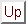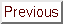Next: A simple direct optimizer: Up: Annexes Previous: Cholesky decomposition.   Contents

# QR factorization

There is another matrix factorization that is sometimes very useful, the so-called QR decomposition,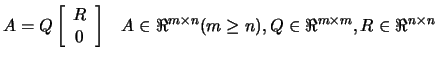(13.44)

Here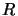is upper triangular, while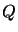is orthogonal, that is,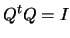(13.45)

where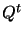is the transpose matrix of. The standard algorithm for the QR decomposition involves successive Householder transformations. The Householder algorithm reduces a matrix A to the triangular form R byorthogonal transformations. An appropriate Householder matrix applied to a given matrix can zero all elements in a column of the matrix situated below a chosen element. Thus we arrange for the first Householder matrix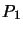to zero all elements in the first column ofbelow the first element. Similarlyzeroes all elements in the second column below the second element, and so on up to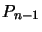. The Householder matrixhas the form: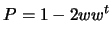(13.46)

whereis a real vector with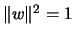. The matrixis orthogonal, because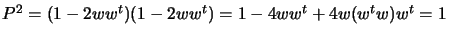. Therefore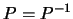. But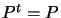, and so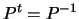, proving orthogonality. Let's rewriteas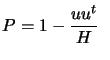with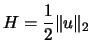(13.47)

andcan now be any vector. Suppose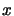is the vector composed of the first column of. Choose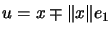where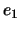is the unit vector, and the choice of signs will be made later. Then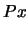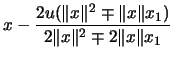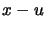This shows that the Householder matrix P acts on a given vectorto zero all its elements except the first one. To reduce a symmetric matrixto triangular form, we choose the vectorfor the first Householder matrix to be the first column. Then the lowerelements will be zeroed: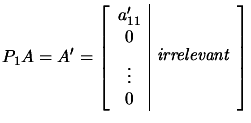(13.48)

If the vectorfor the second Householder matrix is the lowerelements of the second column, then the lower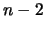elements will be zeroed:(13.49)

Whereand the quantity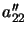is simply plus or minus the magnitude of the vector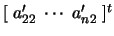. Clearly a sequence ofsuch transformation will reduce the matrix A to triangular form. Instead of actually carrying out the matrix multiplications in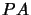, we compute a vector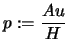. Then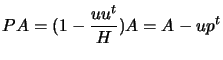. This is a computationally useful formula. We have the following: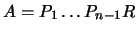. We will thus formby recursion after all the's have been determined: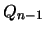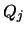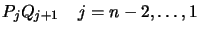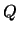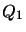No extra storage is needed for intermediate results but the original matrix is destroyed.Next: A simple direct optimizer: Up: Annexes Previous: Cholesky decomposition.   Contents
Frank Vanden Berghen 2004-04-19